# SIMD Programmingin JavaScript*

### May 22, 2014

Peter Jensen (@kaptajnjensen)

Intel® Corporation

Birds
Mandelbrot

## SIMD: Single Instruction, Multiple Data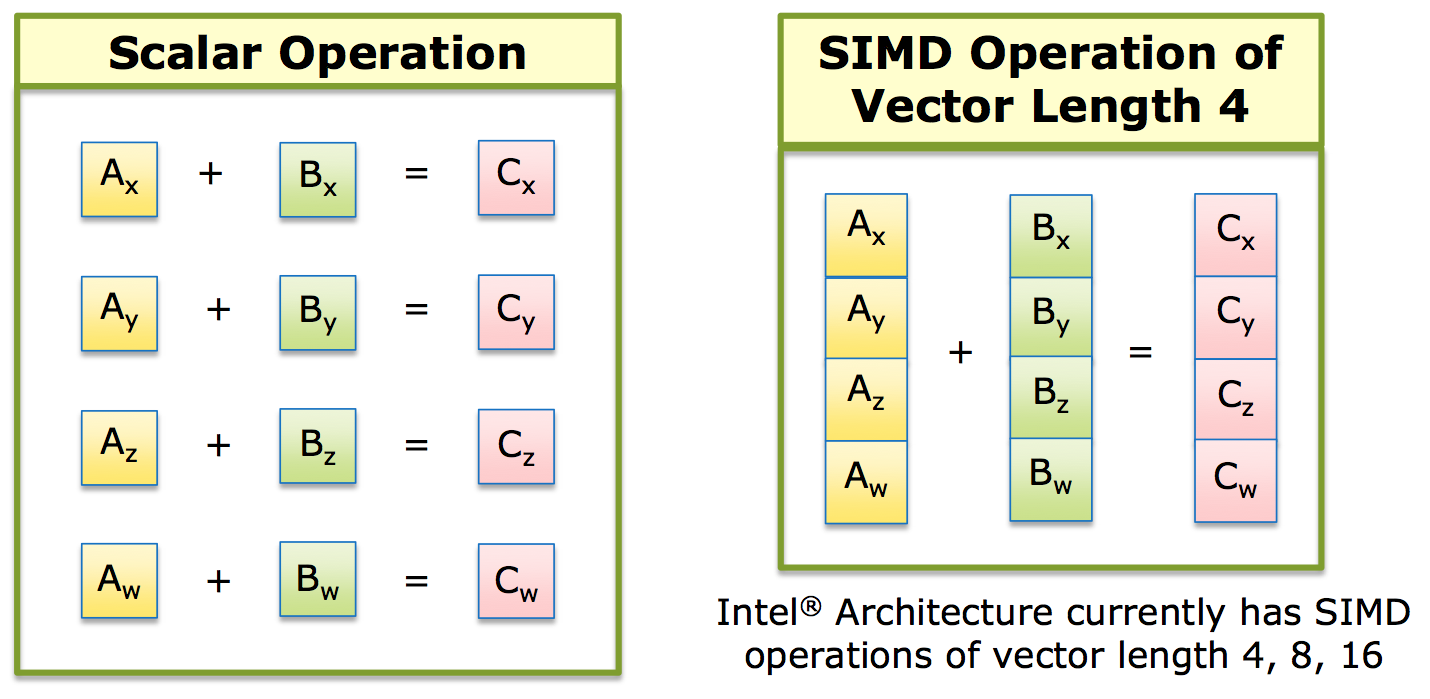## Brief History

• Started mid-2013
• Initial polyfill spec by John McCutchan (Google*/Dart* VM team)
• Prototypes for Chromium*, Firefox*, and Crosswalk

## JavaScript*'s Popularity and Use on the Rise!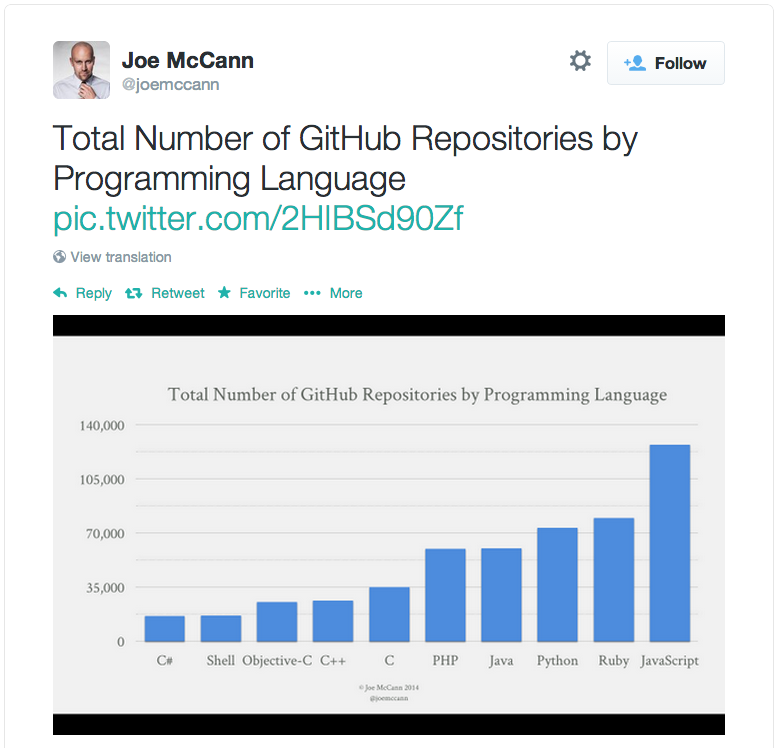Games (Unreal*, Unity*) (via Emscripten*/asm.js) Hybrid HTML5 apps on mobile devices Pure HTML5 apps in ChromeOS*/FirefoxOS*/Tizen* Standalone desktop apps via node-webkit Full featured productivity web apps (Google* Docs, Maps, Intel® XDK etc) Server side logic via node.js

## Hardware/Software Disconnect!

SIMD instructions are an increasingly larger
portion of instruction set architectures of newer CPUs

Currently, no way to utilize these powerful instructions
from JavaScript* programs

## SIMD Programming in C/C++

float average(float *src, int len) {
float sum = 0.0;
for (int i = 0; i < len; ++i) {
sum = sum + src[i];
}
return sum/len;
}

#if defined(__i386__)
float simdAverage(float *src, int len) {
__m128 sumx4 = _mm_setzero_ps();
for (int i = 0; i < len; i += 4) {
src += 4;
}
float sumx4_mem;
_mm_storeu_ps(sumx4_mem, sumx4);
return (sumx4_mem + sumx4_mem +
sumx4_mem + sumx4_mem)/len;
}
#elif defined(__arm__)
float simdAverage(float *src, int len) {
float32x4_t sumx4 = vdupq_n_f32(0.0);
for (int i = 0; i < len; i += 4) {
src += 4;
}
return (vgetq_lane_f32(sumx4,0) + vgetq_lane_f32(sumx4,1) +
vgetq_lane_f32(sumx4,2) + vgetq_lane_f32(sumx4,3))/len;
}
#else
float simdAverage(float *src, int len) {
return average(src, len);
}
#endif


## SIMD Programming in JavaScript*

function simdAverage(src, len) {
var sumx4 = SIMD.float32x4.splat(0.0);
var srcx4 = new Float32x4Array(src.buffer);
for (var i = 0, n = len/4; i < n; ++i) {
}
return (sumx4.x + sumx4.y + sumx4.z + sumx4.w)/len;
}

• Performance: Equivalent to C/C++
• Shared code for:
• All architectures
• All OSes
• All Browsers

• †) Where SIMD browser support is available

## Physics Example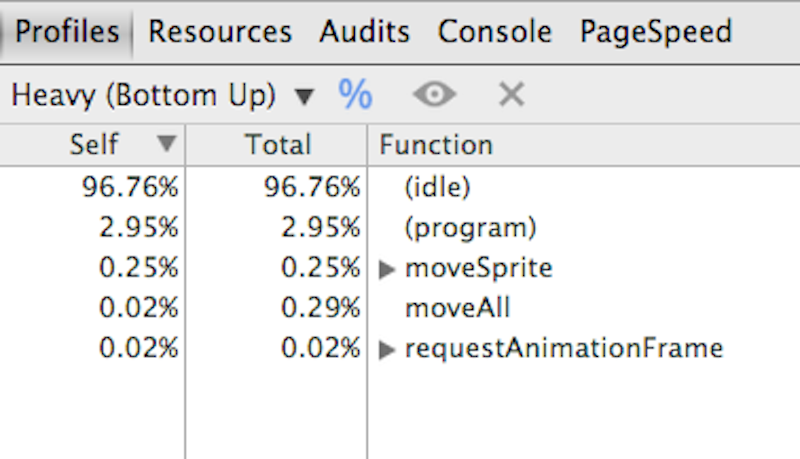## A Little MathConstant Acceleration

${v}_{n+1}=a\Delta t+{v}_{n}$

${s}_{n+1}=\frac{1}{2}a{\left(\Delta t\right)}^{2}+{v}_{n}\Delta t+{s}_{n}$

## Multiple birdsConstant Acceleration

function updateAllConstantAccel(timeDelta) {
var timeDeltaSec = timeDelta/1000.0;
var timeDeltaSecSquared = timeDeltaSec*timeDeltaSec;
for (var i = 0; i < actualBirds; ++i) {
var pos = posArray[i];
var vel = velArray[i];
var newPos = 0.5*accelData.valueConst*timeDeltaSecSquared + vel*timeDeltaSec + pos;
var newVel = accelData.valueConst*timeDeltaSec + vel;
if (newPos > maxPos) {
newVel = -newVel;
}
posArray[i] = newPos;
velArray[i] = newVel;
}
}

## Variable Acceleration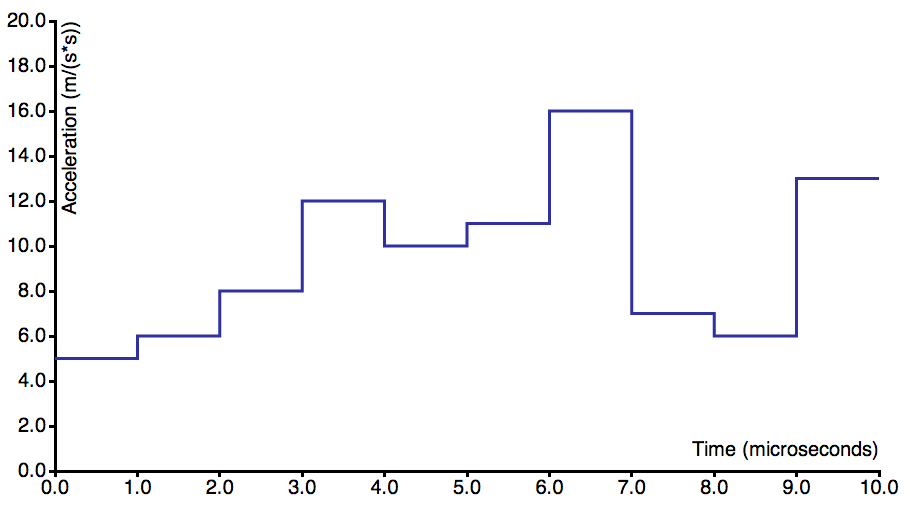## More MathVariable Acceleration

${v}_{n+1}=\left(\sum _{i=0}^{N}{a}_{i}\frac{\Delta t}{N}\right)+{v}_{n}$

${s}_{n+1}=\left(\sum _{i=0}^{N}\frac{1}{2}{a}_{i}{\left(\frac{\Delta t}{N}\right)}^{2}+{v}_{n}\frac{\Delta t}{N}\right)+{s}_{n}$

## Multiple BirdsVariable Acceleration

function updateAllSimd(timeDelta) {
var steps        = accelData.steps;
var accelCount   = accelData.values.length;
var subTimeDelta = timeDelta/steps/1000.0;

var posArrayx4            = new Float32x4Array(posArray.buffer);
var velArrayx4            = new Float32x4Array(velArray.buffer);
var maxPosx4              = SIMD.float32x4.splat(maxPos);
var subTimeDeltax4        = SIMD.float32x4.splat(subTimeDelta);
var subTimeDeltaSquaredx4 = SIMD.float32x4.mul(subTimeDeltax4, subTimeDeltax4);
var point5x4              = SIMD.float32x4.splat(0.5);

for (var i = 0, len = (actualBirds+3)>>2; i < len; ++i) {
var newVelTruex4;
var accelIndex = 0;
var newPosx4   = posArrayx4.getAt(i);
var newVelx4   = velArrayx4.getAt(i);
for (var a = 0; a < steps; ++a) {
var accel   = accelData.values[accelIndex];
var accelx4 = SIMD.float32x4.splat(accel);
accelIndex  = (accelIndex + 1) % accelCount;
var posDeltax4;
posDeltax4   = SIMD.float32x4.mul(point5x4, SIMD.float32x4.mul(accelx4, subTimeDeltaSquaredx4));
var cmpx4    = SIMD.float32x4.greaterThan(newPosx4, maxPosx4);
newVelTruex4 = SIMD.float32x4.neg(newVelx4);
newVelx4     = SIMD.int32x4.select(cmpx4, newVelTruex4, newVelx4);
}
posArrayx4.setAt(i, newPosx4);
velArrayx4.setAt(i, newVelx4);
}
}

## Performance Profiles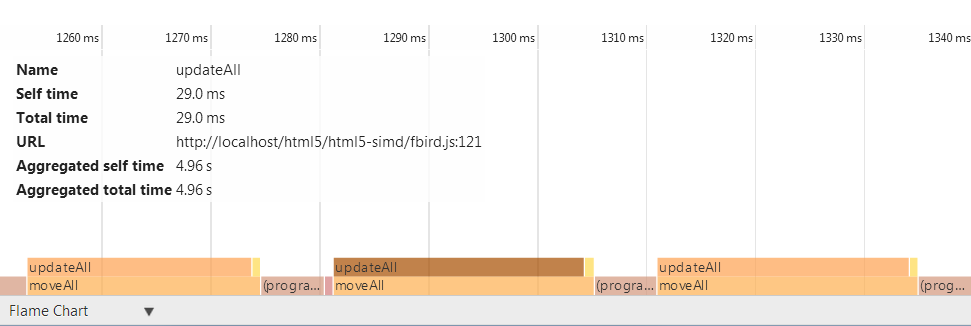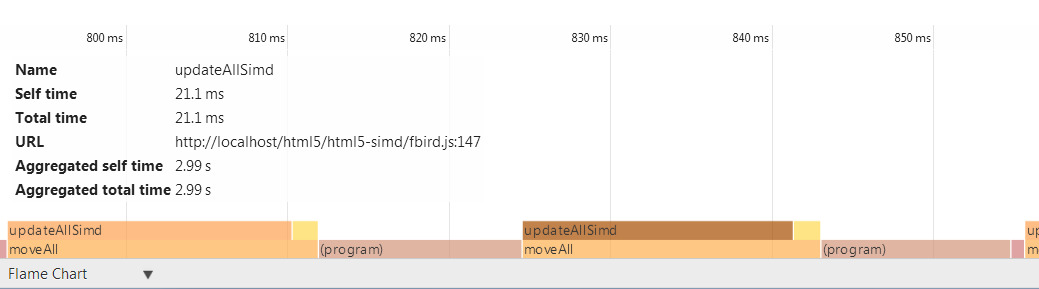## Mandelbrot SIMD Kernel

// z(i+1) = z(i)^2 + c
// terminate when |z|^2 > 4.0
// returns 4 iteration counts
//
function mandelx4(c_re4, c_im4) {
var z_re4  = c_re4,
z_im4  = c_im4,
four4  = SIMD.float32x4.splat (4.0),
two4   = SIMD.float32x4.splat (2.0),
count4 = SIMD.int32x4.splat (0),
one4   = SIMD.int32x4.splat (1),
i, z_re24, z_im24, mi4, new_re4, new_im4;

for (i = 0; i < max_iterations; ++i) {
z_re24 = SIMD.float32x4.mul (z_re4, z_re4);
z_im24 = SIMD.float32x4.mul (z_im4, z_im4);

mi4    = SIMD.float32x4.lessThanOrEqual (SIMD.float32x4.add (z_re24, z_im24), four4);
// if all 4 values are greater than 4.0, there's no reason to continue
break;
}

new_re4 = SIMD.float32x4.sub (z_re24, z_im24);
new_im4 = SIMD.float32x4.mul (SIMD.float32x4.mul (two4, z_re4), z_im4);
count4  = SIMD.int32x4.add (count4, SIMD.int32x4.and (mi4, one4));
}
return count4;
}

## API Details

### Types:

• SIMD.float32x4 : 4 lane 32-bit floats
• SIMD.int32x4 : 4 lane 32 bit ints

### Constructors:

• SIMD.float32x4(x,y,z,w)
• SIMD.int32x4(x,y,z,w)
• .splat(val)
• .zero()

## API Details

### Lane Accessors, Mutators

• Accessors: .x, .y, .z, .w
• Mutators: .withX(), .withY(), .withZ(). withW()

### Operators:

• Arithmetic: .abs() .neg() .add() .sub() .mul() .div()
.reciprocal() reciprocalSqrt() .scale() .sqrt()
• Shuffle: .shuffle() .shuffleMix()
• Logical: .and() .or() .xor() .not()
• Comparison: .equal() .greaterThan() .lessThan()
• Shift: .shiftLeft() .shiftRightLogical()
.shiftRightArithmetic()
• Conversion: .bitsToFloat32x4() .toFloat32x4()
.bitsToInt32x4() .toInt32x4()
• Miscelleneous: .clamp() .min() .max()

## Shuffling - Matrix Transpose

    var src0     = srcx4.getAt(0);
var src1     = srcx4.getAt(1);
var src2     = srcx4.getAt(2);
var src3     = srcx4.getAt(3);

## Shuffling - Matrix Transpose

    tmp01 = SIMD.float32x4.shuffleMix(src0, src1, SIMD.XYXY);
tmp23 = SIMD.float32x4.shuffleMix(src2, src3, SIMD.XYXY);


## Shuffling - Matrix Transpose

    dst0  = SIMD.float32x4.shuffleMix(tmp01, tmp23, SIMD.XZXZ);
dst1  = SIMD.float32x4.shuffleMix(tmp01, tmp23, SIMD.YWYW);

## Shuffling - Matrix Transpose

    dstx4.setAt(0, dst0);
dstx4.setAt(1, dst1);
dstx4.setAt(2, dst2);
dstx4.setAt(3, dst3);

## Prototypes

• Firefox*
Full implementation available internally at Intel®.
Full interpreter implementation has landed in nightly.
Submission of incremental JIT compiler patches is ongoing.
• Chrome*
Full implementation available internally at Intel®.
Patch submitted to Chromium*.
• Crosswalk (Intel®'s open source web runtime, based on Blink*)
Beta version available TODAY!

## Application Domains

• Games:
• Vector/Matrix operations (e.g., glMatrix.js for webGL)
• Physics (e.g., box2D, PhysicsJS)
• Cryptography
• Image/video Processing
• Signal/audio processing/filtering
• Fluid dynamics
• Finance (e.g., Black-Scholes computations)

## Benchmark Results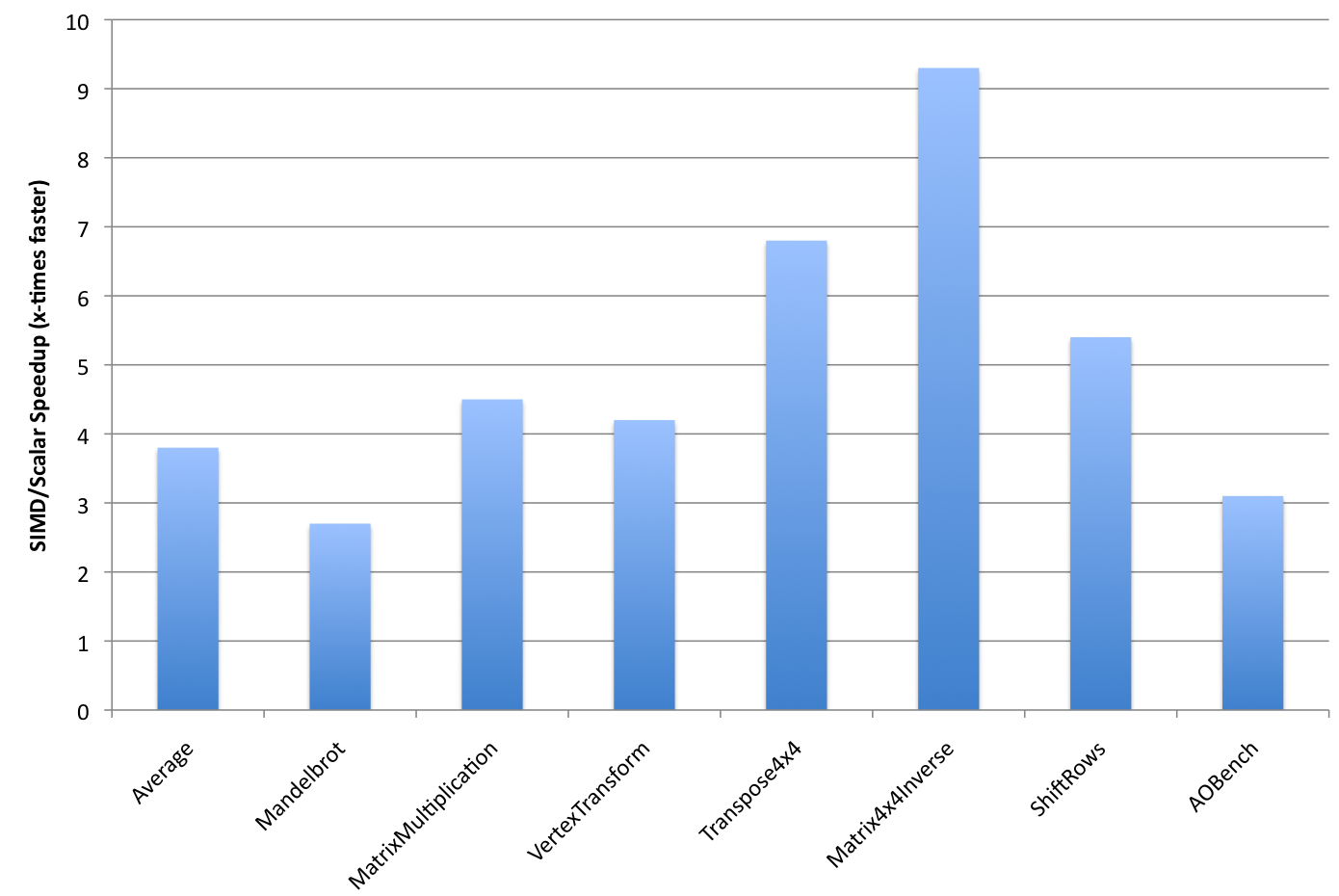Hardware: Intel® Atom processor Z3770 @1.46GHz, Android* 4.4
Benchmark: github.com/johnmccutchan/ecmascript_simd/tree/master/src/benchmarks

## How to use SIMD in JavaScript* TODAY!

Build with Crosswalk Beta## Going Forward

• Complete Firefox* prototype
• Prepare ES7 proposal for July Meeting
• Short Vector Math Library - svml.js (sin/cos/tan/exp/log/...)
• SIMD optimized versions of existing libraries (PhysicsJS, box2DJS, glMatrix.js, etc.)
• Higher level abstraction libraries?

## Acknowledgements

• Mozilla*: Niko Matsakis, Dan Gohman, Luke Wagner, Alon Zakai
• Intel®: Mohammad Haghighat, Ivan Jibaja, Haitao Feng, Ningxin Hu, Weiliang Lin, Heidi Pan

## Thank You!

This presentation: peterjensen.github.io/html5-simd/

## Legal Disclaimer

Intel's compilers may or may not optimize to the same degree for non-Intel microprocessors for optimizations that are not unique to Intel microprocessors. These optimizations include SSE2, SSE3, and SSE3 instruction sets and other optimizations. Intel does not guarantee the availability, functionality, or effectiveness of any optimization on microprocessors not manufactured by Intel.

Microprocessor-dependent optimizations in this product are intended for use with Intel microprocessors. Certain optimizations not specific to Intel microarchitecture are reserved for Intel microprocessors. Please refer to the applicable product User and Reference Guides for more information regarding the specific instruction sets covered by this notice.

Notice revision #20110804